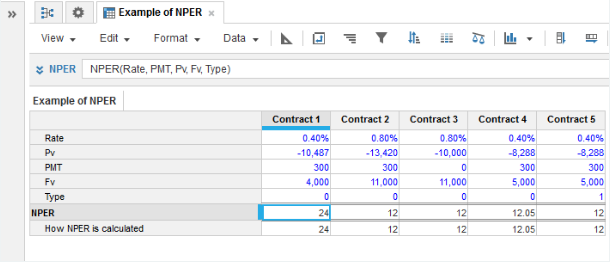1. Calculation functions
2. All Functions
3. Financial Functions
4. NPERNPER is the length in periods of the investment term. That is the number of periodic payments that are needed given the interest rate, opening and closing balances and the payment values required.

Formula:

NPER=
IF Rate =0 THEN (-Pv - Fv)/PMT
ELSE
LOG((-Rate*Fv+PMT*(1+Rate*Type))/(Rate*Pv+PMT*(1+Rate*Type)))/LOG(1+Rate)

where Type = 0 for payments made at the start of the period and Type = 1 for payments made at the end of each period.

## Syntax

NPER(r, [c], p, [f], [t])

where:

• r: Number: Interest rate per period (Leaf-level time periods are treated as of equal length).
• c: Number: Equal payments paid out each period during the course of the investment term. This can be zero, in which case all the interest is compounded and included in the lump sum (Fv) you get at the end. The payments include both an element of interest and an element of capital (optional).
• p: Number: Present value or the initial investment. Cash outflows such as investments are entered as negative numbers. If you are taking out a loan, this is a cash inflow so is entered as a positive number.
• f: Number: Future residual value at the end of the term (optional).
• t: Number: 0 or omitted means payments are made at the start of each period, Type =1 or non-zero means payments are made at the end of each period (optional).

## Format

Input Format Output Format

r: Number: percentage

c: Number: decimal

p: Number: decimal

f: Number: decimal

t: Number: binary

Number: decimal

## Arguments

The function uses the following arguments:

• r: Number: Numeric line item, property, or expression
• c: Number: Numeric line item, property, or expression
• p: Number: Numeric line item, property, or expression
• f: Number: Numeric line item, property, or expression
• t: Number: Numeric line item, property, or expression

## Constraints

The function has no constraints.

## Example

For example, contract 1 has an opening balance of –10487 (the initial investment or Pv) interest set at 0.4% per period and regular payments of 300 per period. To achieve a closing balance of 4000 (Fv), the investment term (NPER) needs to be 24 periods.

NPER(Rate, PMT, Pv, Fv, Type)